# ASCII conversion extension

Extension to convert Ascii code to text and vice versa
AsciiConversion.aix (8.9 KB)

This extension allows user to convert a list of Ascii code to the corresponding text. It also allows to give the Ascii code of a given character and vice versa.

This extension has 3 functions :
**

• AsciiCode

**: This is a function that returns the Ascii code of the character given as a parameter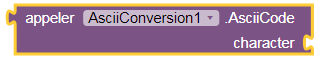Example of use: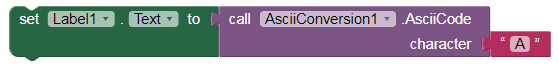This block allows to write in the label 1 the text: "65" which is the Ascii code of the character " A ".
**

• GiveCharacter

**: This is a function that returns the character corresponding to an Ascii code given as a parameter.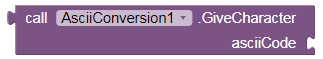Example of use: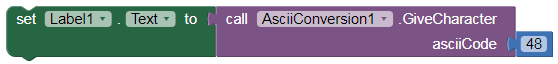This block will write in label 1 the text: "0" which is the character whose Ascii code is "48" .
**

• Conversion

**: It is a function which returns the corresponding text after converting the list of Ascii code given in parameter.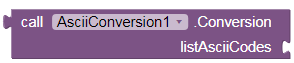This function is very useful when you use the reception of data by UART Bluetooth because the data received by UART is a list containing the Ascii codes that must be converted to the corresponding text. This conversion is ensured by this function
Example of use 1: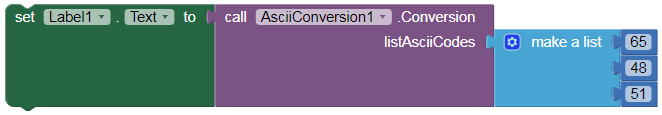This block will write in label 1 the text: "A03" which is the text obtained after the conversion of the Ascii code list [65,48,51] .

Example of use 2: with Bluetooth UART

UART_TX_Field: is a list containing the Ascii codes of the received characters.

If UART_TX_Field is the following list: [51,48] , then the last block will write in the label_temperature the text: "30".

Here is an aia project showing an example of using the extension.
test_AsciiConversion.aia (17.8 KB)

7 Likes

Great extension i like it
This extension solved my problem and its use is very simple

1 Like